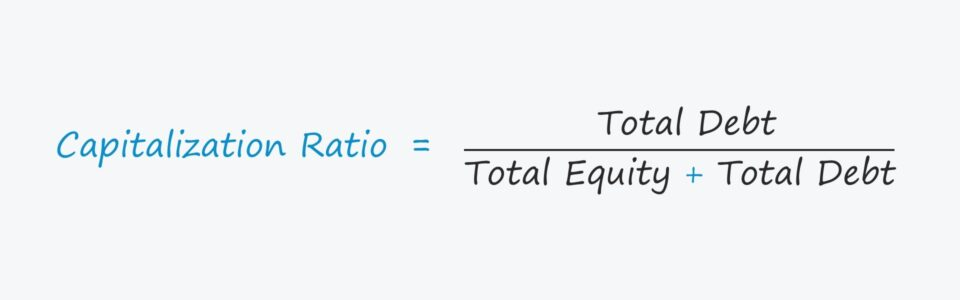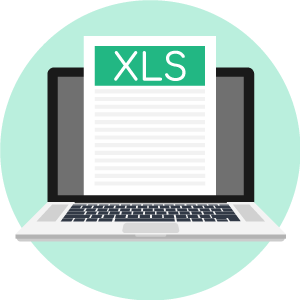# Capitalization Ratio

Guide to Understanding the Capitalization Ratio• What is the definition of the capitalization ratio?
• What formula calculates the capitalization ratio?
• How are the capitalization ratio and credit risk related?
• Would a high or low capitalization ratio be preferred?

## Capitalization Ratio Definition

The capitalization ratio gauges the total amount of debt in a company’s capital structure relative to its two capital sources, equity or debt.

Often referred to as the “debt-to-capital ratio”, the metric compares a company’s total debt to its total capitalization.

Companies generate revenue from their asset base, such as inventory and PP&E, which are purchased using financing from a source of capital. There are typically two main sources of capital:

1. Total Equity: Paid-in-Capital, Retained Earnings, Equity Issuances
2. Total Debt: Senior Debt, Corporate Bonds, Mezzanine Financing

Debt can consist of short-term and long-term borrowings like senior secured loans provided by bank lenders or issuances of corporate bonds.

In comparison, debt financing is considered to be “cheaper” than equity financing because of:

• Tax-Deductible Interest: The pre-tax income is reduced by interest expense on the income statement, as interest is tax-deductible – creating the so-called “interest tax shield”.
• Higher Priority: If the company were to file for bankruptcy and undergo liquidation, the claims held by debt holders are prioritized ahead of that of equity holders in terms of distributing recoveries.

The drawback to debt financing, however, is that there are fixed financing costs, which can lead to a potential default (i.e. missed interest payment, mandatory principal amortization, covenant breach).

Furthermore, the downside to equity issuances is that the additional shares issues can dilute ownership in the company.

## Capitalization Ratio Interpretation

All else being equal, the higher the capitalization ratio, the riskier the company is due to its reliance on financial leverage.

The higher a company’s capitalization ratio, the more its capital structure is implied to be comprised of debt rather than equity.

Therefore, the company is at a greater risk of defaulting and becoming distressed.

By contrast, a lower capitalization ratio – which is viewed more favorably from a credit risk perspective – indicates that the company is less dependent on debt.

The relationship between the capitalization ratio and default risk are as follows:

• Higher Capitalization Ratio → More Default Risk
• Lower Capitalization Ratio → Less Default Risk
###### Limitations to the Capitalization Ratio

The capitalization ratio is insufficient as a standalone metric to understand a company’s true financial health.

For instance, a company can utilize minimal debt because of a lack of access to debt financing, rather than by choice.

A company could also possess a lower capitalization ratio to its peers, yet soon file for bankruptcy later.

One critical consideration when comparing the capitalization ratios among different companies is that the companies with the option to raise significant amounts of debt capital are typically the most financially sound.

Why? Lenders tend to be risk-averse, especially senior lenders like corporate banks, so high-risk companies are going to typically be more limited in being capable of raising debt capital.

## Capitalization Ratio Formula

The capitalization ratio formula consists of dividing a company’s total debt by its total capitalization, i.e the sum of total debt and total equity.

### Cap Ratio Formula

• Capitalization Ratio = Total Debt / (Total Equity + Total Debt)

When attempting to decide which line items qualify as debt, all interest-bearing securities with debt-like characteristics should be included.

However, “Total Debt” should typically consist of exclusively long-term debt.

## Capitalization Ratio vs Debt-to-Equity (D/E) Ratio

Often, certain people use the term “capitalization ratio” interchangeably with the debt-to-equity (D/E) ratio.

• D/E Ratio → Debt-to-Equity
• Capitalization Ratio → Debt-to-Capital

The two credit metrics are similar with both measuring the financial risk attributable to companies. The only distinction is the denominator – but other than that, the insights obtained are largely the same.

If you want to ensure there is no chance of a mishap, one option is to clarify the metric as the “total capitalization ratio”.

## Capitalization Ratio Calculator – Excel Template

We’ll now move to a modeling exercise, which you can access by filling out the form below.Submitting ...

## Capitalization Ratio Example Calculation

Suppose we have three companies with different capital structures.

• Company A: Debt = \$25 million; Equity = \$25 million
• Company B: Debt = \$50 million; Equity = \$25 million
• Company C: Debt = \$25 million; Equity = \$50 million

Using those assumptions, the capitalization ratio for each company can be calculated by dividing the total debt by the total capitalization (total debt + total equity).

• Capitalization Ratio = Total Debt ÷ Total Capitalization

We arrive at the following capitalization ratios.

• Company A = \$25 million ÷ (\$25 million + \$25 million) = 0.5x
• Company B = \$50 million ÷ (\$25 million + \$50 million) = 0.7x
• Company C = \$25 million ÷ (\$50 million + \$25 million) = 0.3x

Given the capitalization ratios of the group, it appears Company C carries the least leverage risk while Company B is the riskiest of the three.

Just as a general rule of thumb, if the cap ratio is less than 0.5x, then the company would be considered as financially stable with minimal risk of default.

However, as mentioned earlier, the capitalization ratio must also be supported by other credit metrics to confirm the validity of the findings.Inline FeedbacksLearn Financial Modeling Online

Everything you need to master financial and valuation modeling: 3-Statement Modeling, DCF, Comps, M&A and LBO.

X

The Wall Street Prep Quicklesson Series

7 Free Financial Modeling Lessons

Get instant access to video lessons taught by experienced investment bankers. Learn financial statement modeling, DCF, M&A, LBO, Comps and Excel shortcuts.